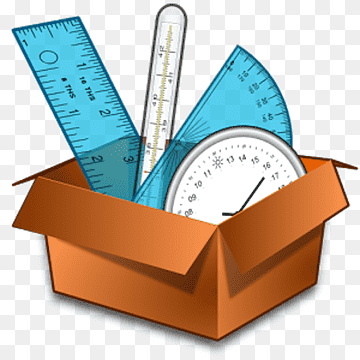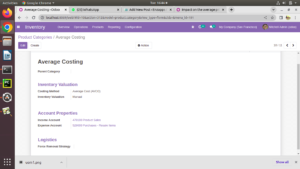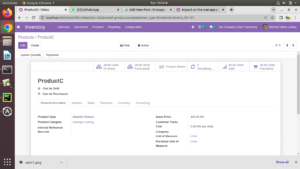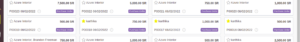## How to Configure Average Costing

The average cost method calculates the cost of ending inventory and cost of goods sold on the basis of weighted average cost per unit of inventory.

Now first you need to add average cost in your product category: inventory ->configuration ->product category ->create average cost, in that you need to set costing method :Average Cost(AVCO).Create  a new product with category:Average CostCreate two or more purchase quotation .At the beginning, the Avg Cost is set to 0 set as there is no product in the inventory.When the first reception is made, the average cost becomes logically the purchase price.At the second reception, the average cost is updated .

Here the average cost will be : 75 per unit